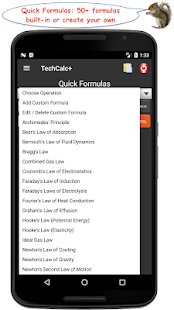A calculator with 10 computing modes in one application + a handy scientific reference facility – different modes allow: 1) basic arithmetic (both decimals and fractions), 2) scientific calculations, 3) hex, oct & bin format calculations, 4) graphing applications, 5) matrices, 6) complex numbers, 7) quick formulas (including the ability to create custom formulas),quick conversions, 9) solving algebraic equations & 10) time calculations.
Please note that internet permission is needed to allow access to currency exchange rates in the conversion function
Functions include:

• General Arithmetic Functions
• Trigonometric Functions – radians, degrees & gradients – including hyperbolic option
• Power & Root Functions
• Log Functions
• Modulus Function
• Random Number Functions
• Permutations (nPr) & Combinations (nCr)
• Highest Common Factor & Lowest Common Multiple
• Statistics Functions – Statistics Summary (returns the count (n), sum, product, sum of squares, minimum, maximum, median, mean, geometric mean, variance, coefficient of variation & standard deviation of a series of numbers), Bessel Functions, Beta Function, Beta Probability Density, Binomial Distribution, Chi-Squared Distribution, Confidence Interval, Digamma Function, Error Function, Exponential Density, Fisher F Density, Gamma Function, Gamma Probability Density, Hypergeometric Distribution, Normal Distribution, Poisson Distribution, Student T-Density & Weibull Distribution
• Conversion Functions – covers all common units for distance, area, volume, weight, density, speed, pressure, energy, power, frequency, magnetic flux density, dynamic viscosity, temperature, heat transfer coefficient, time, angles, data size, fuel efficiency & exchange rates
• Constants – a wide range of inbuilt constants listed in 4 categories:
1) Physical & Astronomical Constants – press to include into a calculation or long press for more information on the constant and its relationship to other constants
2) Periodic Table – a full listing of the periodic table – press to input an element’s atomic mass into a calculation or long press for more information on the chosen element – the app also includes a clickable, pictorial representation of the periodic table
3) Solar System – press to input a planet’s orbit distance into a calculation or long press for more information on the chosen planet
4) My Constants – a set of personal constants that can be added via the History
• Convert between hex, oct, bin & dec
• AND, OR, XOR, NOT, NAND, NOR & XNOR Functions
• Left Hand & Right Hand Shift
• Plotter with a table also available together with the graph
• Complex numbers in Cartesian, Polar or Euler Identity format
• The main screen of the calculator can also be set to Fractions Mode for general arithmetic functions including use of parentheses, squares, cubes and their roots
• 20 Memory Registers in each of the calculation modes
• A complete record of each calculation is stored in the calculation history, the result of which can be used in future calculations
An extensive help facility is available which also includes some useful scientific reference sections covering names in the metric system, useful mathematical formulas and a detailed listing of physical laws containing a brief description of each law.
A default screen layout is available for each function showing all buttons on one screen or, alternatively, all the functions are also available on a range of scrollable layouts which are more suitable for small screens – output can be set to scroll either vertically (the default) or horizontally as preferred – output font size can be increased or decreased by long pressing the + or – buttons
A full range of settings allow easy customisation – move to SD for 2.2+ users
Please email any questions that are not answered in the help section or any requests for bug fixes, changes or extensions regarding the functions of the calculator – glad to help wherever possible.

WHAT’S NEW

18/10 (ver 4.0.1)
* bug fix in constants (when creating a custom formula) inadvertently introduced in the previous update
05/10 (ver 4.0.0)
* added Integer Sequences (Fibonacci Numbers, Catalan Numbers and Polygonal Numbers) to the Reference Section
* Calculus (Derivative and Indefinite Integral) – after the calculation, long press the output display to show a graph of the input and result

Screenshot :

•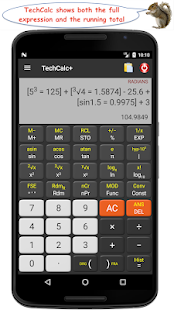•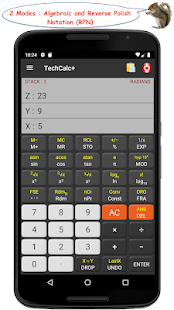•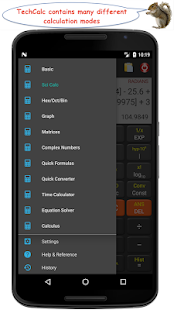••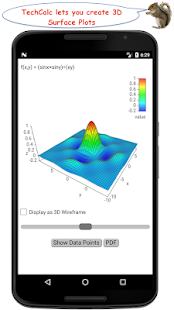•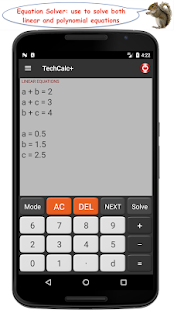•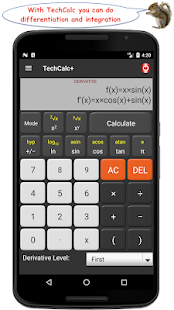•